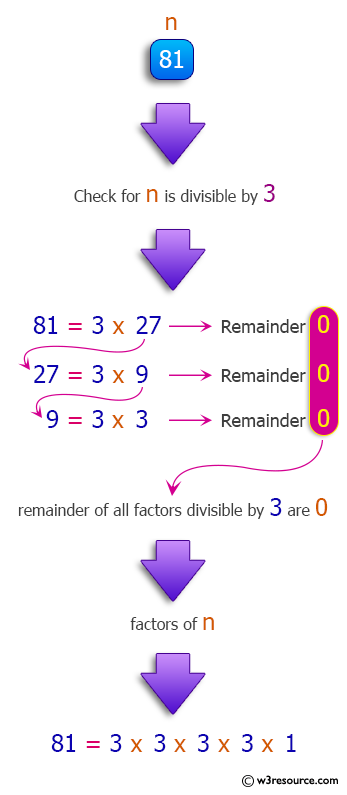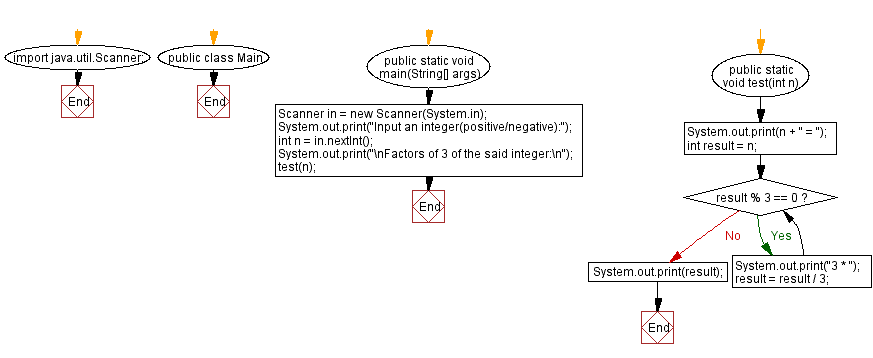﻿ Java - Display the factors of 3 in a given integer

# Java: Display the factors of 3 in a given integer

## Java Method: Exercise-21 with Solution

Write a Java method to display the factors of 3 in a given integer.

Pictorial Presentation:Sample data:
(8) -> 8 = 8
(45) -> 45 = 3 * 3 * 5
(81) -> 81 = 3 * 3 * 3 * 3 * 1

Sample Solution:

Java Code:

``````import java.util.Scanner;
public class Main {
public static void main(String[] args)
{
Scanner in = new Scanner(System.in);
System.out.print("Input an integer(positive/negative):");
int n = in.nextInt();
System.out.print("\nFactors of 3 of the said integer:\n");
test(n);
}

public static void test(int n){
System.out.print(n + " = ");
int result = n;
while (result % 3 == 0){
System.out.print("3 * ");
result = result / 3;
}
System.out.print(result);
}
}
```
```

Sample Output:

```Input an integer(positive/negative): 81

Factors of 3 of the said integer:
81 = 3 * 3 * 3 * 3 * 1
```

Flowchart :Java Code Editor: